# Surface Areas and Volumes (Mathematics) Class 9 - NCERT Questions

Q 1.

A plastic box 1.5 m long, 1.25 m wide and 65 cm deep is to be made. It is opened at the top. Ignoring the thickness of the plastic sheet, determine:
(i) The area of the sheet required for making the box.
(ii) The cost of sheet for it, if a sheet measuring 1 m² costs ₹ 20.

SOLUTION:

(i) Here, l=1.5 m, b=1.25 m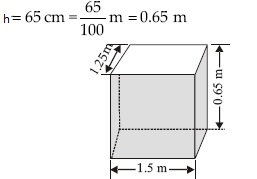∴ It is open from the top.
∴ Its surface area
= [Lateral surface area] + [Base area]=[2(l + b)h] + [lb]
= [2(1.50 + 1.25)0.65] m² + [1.50 x 1.25] m²
= [2 x 2.75 x 0.65] m² + [1.875] m²
= 3.575 m² + 1.875 m²=5.45 m²
∴ Area of the sheet required for making the box=5.45 m²
(ii) Cost of 1 m² sheet=₹ 20
∴ Cost of 5.45 m² sheet=₹ (20 x 5.45)
= ₹ 109
∴ Cost of the required sheet=₹ 109

Q 2.

The length, breadth and height of a room are 5 m, 4 m and 3 m respectively. Find the cost of white washing the walls of the room and the ceiling at the rate of ₹7.50 per m².

SOLUTION:

Length of the room (l)=5 m
Breadth of the room (b)=4 m
Height of the room (h)=3 m
The room is like a cuboid whose four walls (lateral surface) and ceiling are to be white washed.
∴ Area for white washing
= [Lateral surface area] + [Area of the ceiling]
= [2(l + b)h] + [l x b]
= [2(5 + 4) x 3] m² + [5 x 4] m²
=  m² +  m²=74 m²
Cost of white washing for 1 m² area=₹ 7.50
∴ Cost of white washing for 74 m² area=₹ (7.50 x 74)=₹ 555
∴ The required cost of white washing= ₹ 555

Q 3.

The floor of a rectangular hall has a perimeter 250 m. If the cost of painting the four walls at the rate of ₹ 10 per m² is ₹ 15000, find the height of the hall.
[Hint: Area of the four walls=Lateral surface area]

SOLUTION:

A rectangular hall means a cuboid.
Let the length and breadth of the hall be l and b respectively.
∴ Perimeter of the floor=2(l + b)
∴ 2(l + b)=250 m
∴ Area of four walls=Lateral surface area
= 2(l + b) x h, where h is the height of hall
∴ Cost of painting the four walls
= ∴ (10 x 250 h)=∴ 2500 h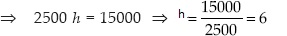Thus, the required height of the hall=6 m

Q 4.

The paint in a certain container is sufficient to paint an area equal to 9.375 m². How many bricks of dimensions 22.5 cm x 10 cm x 7.5 cm can be painted out of this container?

SOLUTION:

Total area that can be painted=9.375 m², Since a brick is like a cuboid, then
total surface area of a brick=2[lb + bh + hl]
= 2[(22.5 x 10) + (10 x 7.5) + (7.5 x 22.5)] cm²
= 2[(225) + (75) + (168.75)] cm²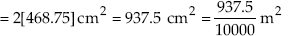Let the required number of bricks=n
∴ Total surface area of n bricks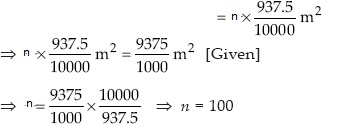Thus, the required number of bricks=100

Q 5.

A cubical box has each edge 10 cm and another cuboidal box is 12.5 cm long, 10 cm wide and 8 cm high.
(i) Which box has the greater lateral surface area and by how much.
(ii) Which box has the smaller total surface area and by how much.

SOLUTION:

For the cubical box with edge=10 cm
Lateral surface area = 4a2
= 4 x 102 cm² = 4 x 100 cm² = 400 cm²
Total surface area = 6a2
= 6 x 102 = 6 x 100 cm² = 600 cm²
For the cuboidal box with dimensions
l=12.5 cm, b=10 cm, h=8 cm
∴ Lateral surface area=2[l + b] x h
= 2[12.5 + 10] x 8 cm²=360 cm² ∴ Total surface area=2[lb + bh + hl]
= 2[(12.5 x 10) + (10 x 8) + (8 x 12.5)] cm²
= 2[125 + 80 + 100] cm²
= 2 cm²=610 cm²
(i) Cubical box has the greater lateral surface area by 400 - 360=40 cm²
. (ii) Total surface area of cubical box is smaller than the cuboidal box by
610 - 600=10 cm².

Q 6.

A small indoor greenhouse (herbarium) is made entirely of glass panes (including base) held together with tape. It is 30 cm long, 25 cm wide and 25 cm high.
(i) What is the area of the glass.
(ii) How much of tape is needed for all the 12 edges.

SOLUTION:

The herbarium is like a cuboid
Here, l=30 cm, b=25 cm, h=25 cm
(i) Surface area of a cuboid=2[lb + bh + hl]
∴ Surface area of the herbarium (glass)
= 2[(30 x 25) + (25 x 25) + (25 x 30)] cm²
= 2[750 + 625 + 750] cm²
= 2 cm²=4250 cm²
Thus, the required area of glass is 4250 cm²
(ii) Total length of 12 edges=4l + 4b + 4h
= 4(l + b + h)=4(30 + 25 + 25) cm
= 4 x 80 cm=320 cm
Thus, required length of tape=320 cm

Q 7.

Shanti Sweets Stall was placing an order for making cardboard boxes for packing their sweets. Two sizes of boxes were required. The bigger of dimensions
25 cm x 20 cm x 5 cm and the smaller of dimensions 15 cm x 12 cm x 5 cm. For all the overlaps, 5% of the total surface area is required extra. If the cost of the cardboard is ₹ 4 for 1000 cm²,
find the cost of cardboard required for supplying 250 boxes of each kind.

SOLUTION:

For bigger box:
Length (l)=25 cm, Breadth (b)=20 cm,
Height (h)=5 cm
Total surface area of a box
= 2(lb + bh + hl)
= 2[(25 x 20) + (20 x 5) + (5 x 25)] cm²
= 2[500 + 100 + 125] cm²
= 2 cm²=1450 cm²
∴ Total surface area of 250 boxes
= (250 x 1450) cm²=362500 cm²
For smaller box:
l=15 cm, b=12 cm, h=5 cm
Total surface area of a box=2[lb + bh + hl]
= 2[(15 x 12) + (12 x 5) + (5 x 15)] cm²
= 2[180 + 60 + 75] cm²=2 cm²=630 cm²
∴ Total surface area of 250 boxes
= 250 x 630 cm²=157500 cm²
Now, total surface area of both type of boxes
= 362500 cm² + 157500 cm²=520000 cm²
Area for overlaps=5% of [total surface area]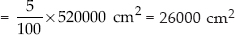∴ Total surface area of the cardboard required
[Total surface area of 250 boxes of each type] + [5% of total surface area]
= 520000 cm² + 26000 cm²=546000 cm²
Cost of 1000 cm² cardboard=₹ 4
∴ Cost of 546000 cm² cardboard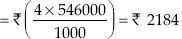Q 8.

Parveen wanted to make a temporary shelter for her car, by making a box-like structure with tarpaulin that covers all the four sides and the top of the car (with the front face as a flap which
can be rolled up). Assuming that the stitching margins are very small, and therefore negligible, how much tarpaulin would be required to make the shelter of height 2.5 m, with base dimensions
4 m x 3 m.

SOLUTION:

Here, height (h)=2.5 m
Base dimension=4 m x 3 m
∴ Length (l)=4 m and breadth (b)=3 m
The surface is like a cuboid
∴ The surface area of the cuboid, excluding the base
=[Lateral surface area] + [Area of ceiling]
= [2(l + b)h] + [lb]
= [2(4 + 3) x 2.5] m² + [4 x 3] m²
=  m² +  m²=47 m²
Thus, 47 m² tarpaulin would be required.

Q 9.

The curved surface area of a right circular cylinder of height 14 cm is 88 cm². Find the diameter of the base of the cylinder.

SOLUTION:

Let r be the radius of the cylinder.
Here, height (h)=14 cm and curved surface area=88 cm²
Curved surface area of a cylinder=2πrh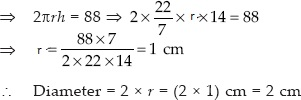Q 10.

It is required to make a closed cylindrical tank of height 1 m and base diameter 140 cm from a metal sheet. How many square metres of the sheet are required for the same.

SOLUTION:

Here, height (h)=1 m
Diameter of the base=140 cm=1.40 m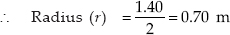Total surface area of the cylinder=2rπ(h + r)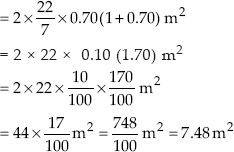Hence, the required sheet is 7.48 m²

Q 11.

A metal pipe is 77 cm long. The inner diameter of a cross section is 4 cm, the outer diameter being 4.4 cm (see fig.). Find its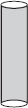(i) inner curved surface area,
(ii) outer curved surface area,
(iii) total surface area.

SOLUTION:

Length of the metal pipe=77 cm
It is in the form of a cylinder
∴ Height (h) of the cylinder=77 cm
Inner diameter=4 cm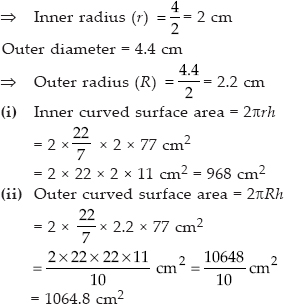(iii) Total surface area=[Inner curved surface area] + [Outer curved surface area]
+ [Area of two circular ends]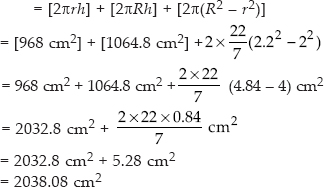Q 12.

The diameter of a roller is 84 cm and its length is 120 cm. It takes 500 complete revolutions to move once over to level a playground. Find the area of the playground in m².

SOLUTION:

The roller is in the form of a cylinder of diameter=84 cm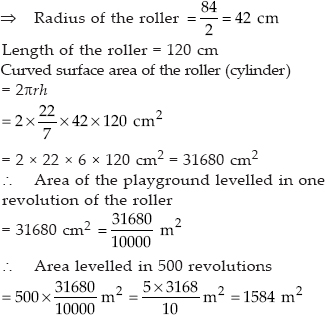Q 13.

A cylindrical pillar is 50 cm in diameter and 3.5 m in height. Find the cost of painting the curved surface of the pillar at the rate of ₹ 12.50 per m².

SOLUTION:

Diameter of the pillar=50 cm,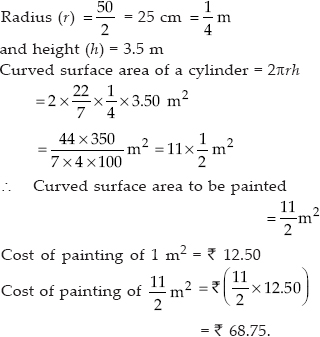Q 14.

Curved surface area of a right circular cylinder is 4.4 m². If the radius of the base of the cylinder is 0.7 m, find its height.

SOLUTION:

Let height of the cylinder be h metres.
Curved surface area of a cylinder=2πrh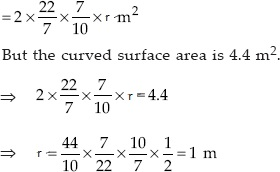Thus, the required height is 1 metre.

Q 15.

The inner diameter of a circular well is 3.5 m. It is 10 m deep. Find
(i) its inner curved surface area.
(ii) the cost of plastering this curved surface at the rate of ₹ 40 per m².

SOLUTION:

Inner diameter of the well=3.5 m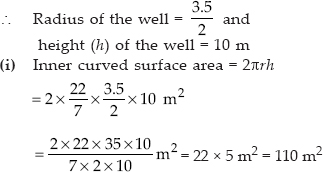(ii) Cost of plastering per m²=₹ 40
Therefore, Total cost of plastering the area 110 m²
= ₹ (110 x 40)=₹ 4400

Q 16.

In a hot water heating system, there is a cylindrical pipe of length 28 m and diameter 5 cm. Find the total radiating surface in the system.

SOLUTION:

Length of the cylindrical pipe=28 m
i.e., h=28 m
Diameter of the pipe=5 cm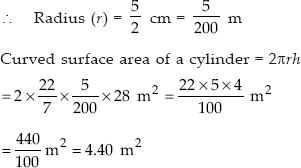Thus, the total radiating surface is 4.40 m²

Q 17.

Find:
(i) the lateral or curved surface area of a closed cylindrical petrol storage tank that is 4.2 m in diameter and 4.5 m high.
(ii) how much steel was actually used, if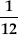of the steel actually used was wasted in making the tank.

SOLUTION:

The storage tank is in the form of a cylinder
∴ Diameter of the tank=4.2 m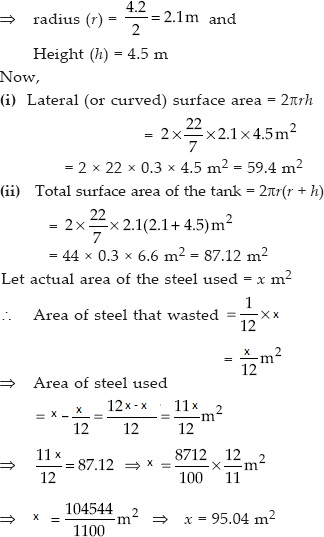Thus, the required area of the steel that was actually used is 95.04 m²

Q 18.

In the figure, you see the frame of a lampshade. It is to be covered with a decorative cloth. The frame has a base diameter of 20 cm and height of 30 cm. A margin of 2.5 cm is to be given for
folding it over the top and bottom of the frame. Find how much cloth is required for covering the lampshade.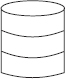SOLUTION:

The lampshade is in the form of a cylinder,
where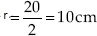and Height=30 cm.
A margin of 2.5 cm is to be added to top and bottom
∴ Total height of the cylinder, h
= 30 cm + 2.5 cm + 2.5 cm=35 cm
Now, curved surface area=2πrh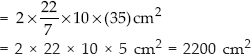Thus, the required area of the cloth
= 2200 cm²

Q 19.

The students of a Vidyalaya were asked to participate in a competition for making and decorating penholders in the shape of a cylinder with a base, using cardboard.
Each penholder was to be of radius 3 cm and height 10.5 cm. The Vidyalaya was to supply the competitors with cardboard. If there were 35 competitors,
how much cardboard was required to be bought for the competition.

SOLUTION:

Here, the penholders are in the form of cylinders
Radius of a cylinder (r)=3 cm
Height of a cylinder (h)=10.5 cm
Since, a penholder must be open from the top
Now, Surface area of a penholder (cylinder)
= [Lateral surface area] + [Base area]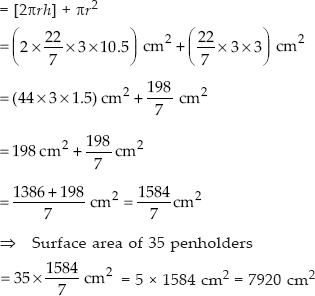Thus, 7920 cm² of cardboard was required to be bought.

Q 20.

Diameter of the base of a cone is 10.5 cm and its slant height is 10 cm. Find its curved surface area.

SOLUTION:

Here, diameter of the base=10.5 cm and Slant height (l)=10 cm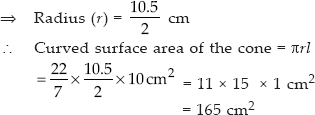Q 21.

Find the total surface area of a cone, if its slant height is 21 m and diameter of its base is 24 m.

SOLUTION:

Here, diameter=24 m and
Slant height (l)=21 m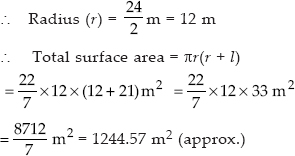Q 22.

Curved surface area of a cone is 308 cm² and its slant height is 14 cm. Find
(i) radius of the base and
(ii) total surface area of the cone.

SOLUTION:

Here, curved surface area=308 cm²
Slant height (l)=14 cm
(i) Let the radius of the base be r cm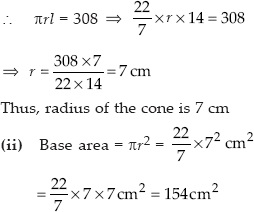and curved surface area=308 cm² [Given]
∴ Total surface area=[Curved surface area] + [Base area]
= 308 cm² + 154 cm²=462 cm²

Q 23.

A conical tent is 10 m high and the radius of its base is 24 m. Find
(i) slant height of the tent.
(ii) cost of the canvas required to make the tent, if the cost of 1m² canvas is ₹70.

SOLUTION:

Here, height of the tent (h)=10 m
Radius of the base (r)=24 m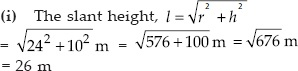Thus, the required slant height of the tent is 26 m
(ii) Curved surface area of the cone=rl
∴ Area of the canvas required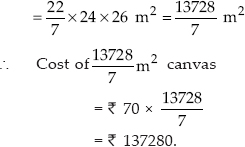Q 24.

What length of tarpaulin 3 m wide will be required to make conical tent of height 8 m and base radius 6 m∴ Assume that the extra length of material that will be required for stitching margins
and wastage in cutting is approximately 20 cm (Use Z= 3.14).

SOLUTION:

Here, Base radius (r)=6 m; Height (h)=8 mThus, area of the canvas (tarpaulin) required to make the tent=188.4 m²
Let the length of the tarpaulin=L m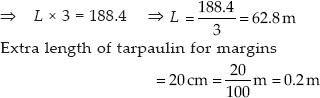Thus, total length of tarpaulin required=62.8 m + 0.2 m=63 m

Q 25.

The slant height and base diameter of a conical tomb are 25 m and 14 m respectively. Find the cost of white-washing its curved surface at the rate of ₹ 210 per 100 m².

SOLUTION: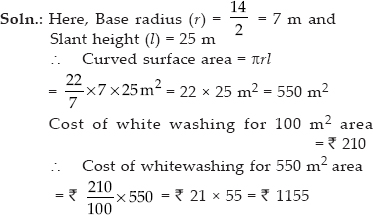Q 26.

A joker's cap is in the form of a right circular cone of base radius 7 cm and height 24 cm. Find the area of the sheet required to make 10 such caps.

SOLUTION:

Radius of the base (r)=7 cm and
height (h)=24 cm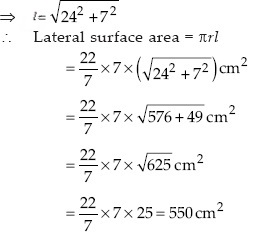∴ Lateral surface area of 10 caps
= 10 x 550 cm²=5500 cm²
Thus, the required area of the sheet
= 5500 cm²

Q 27.

A bus stop is barricaded from the remaining part of the road, by using 50 hollow cones made of recycled cardboard. Each cone has a base diameter of 40 cm and height 1 m.
If the outer side of each of the cones is to be painted and the cost of painting is ₹ 12 per m², what will be the cost of painting all these cones∴ (Use Z= 3.14 and take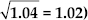SOLUTION:

Diameter of the base=40 cm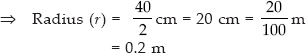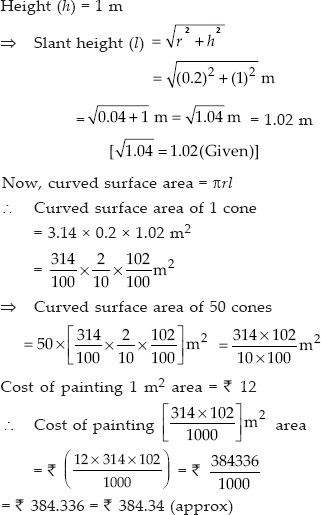Thus, the required cost of painting is ₹ 384.34 (approx).

Q 28.

Find the surface area of a sphere of radius:
(i) 10.5 cm
(ii) 5.6 cm
(iii) 14 cm

SOLUTION:

(i) Here, r=10.5 cm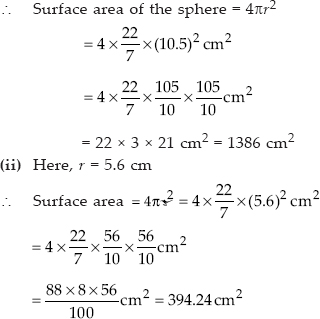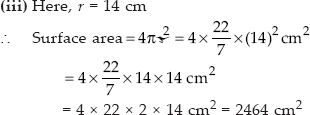Q 29.

Find the surface area of a sphere of diameter
(i) 14 cm
(ii) 21 cm
(iii) 3.5 m

SOLUTION:

(i) Here, Diameter=14 cm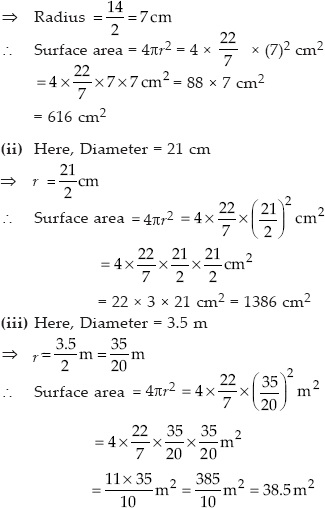Q 30.

Find the total surface area of a hemisphere of radius 10 cm. (Use π= 3.14)

SOLUTION:

Total surface area of hemisphere=3r²
= 3 x 3.14 x 10 x 10 cm²=942 cm²

Q 31.

The radius of a spherical balloon increases from 7 cm to 14 cm as air is being pumped into it. Find the ratio of surface areas of the balloon in the two cases.

SOLUTION:

Case I : When radius (r1)=7 cm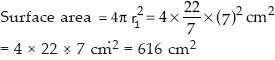Case II : When radius (r²)=14 cm
Surface area=4 x 22 x 14 x 2 cm²=2464 cm²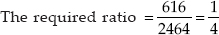Hence, the required ratio=1 : 4.

Q 32.

A hemispherical bowl made of brass has inner diameter 10.5 cm. Find the cost of tin-plating it on the inside at the rate of ₹ 16 per 100 cm².

SOLUTION:

Inner diameter of the hemisphere=10.5 cm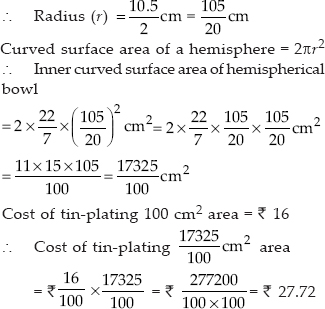Q 33.

Find the radius of a sphere whose surface area is 154 cm².

SOLUTION:

Let the radius of the sphere be r cm.
Surface area = 4r²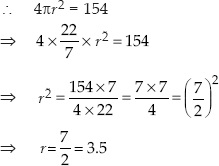Thus, the required radius of the sphere is 3.5 cm.

Q 34.

The diameter of the moon is approximately one fourth of the diameter of the earth. Find the ratio of their surface areas.

SOLUTION:

Let the radius of the earth=r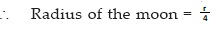Surface area of a sphere=4r²
Since, the earth as well as the moon are considered to be spheres
∴ Surface area of the earth=4r²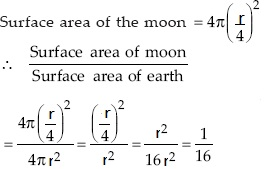Thus, the required ratio=1 : 16.

Q 35.

A hemispherical bowl is made of steel, 0.25 cm thick. The inner radius of the bowl is 5 cm. Find the outer curved surface area of the bowl.

SOLUTION: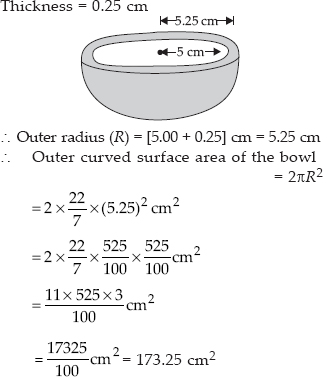Q 36.

A right circular cylinder just encloses a sphere of radius r (see fig.). Find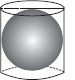(i) surface area of the sphere,
(ii) curved surface area of the cylinder,
(iii) ratio of the areas obtained in (i) and (ii).

SOLUTION:

(i) For the sphere: Radius = r
∴ Surface area of the sphere = 4πr²
(ii) For the right circular cylinder:
∴ Radius of the cylinder = r
Height of the cylinder = Diameter of the sphere
∴ Height of the cylinder (h) = 2r
Since, curved surface area of a cylinder = 2πrh = 2πr(2r) = 4πr²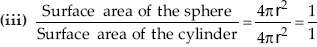Thus, the required ratio is 1 : 1.

Q 37.

A matchbox measures 4 cm x 2.5 cm x 1.5 cm. What will be the volume of a packet containing 12 such boxes∴

SOLUTION:

Measures of matchbox (cuboid) is 4 cm x 2.5 cm x 1.5 cm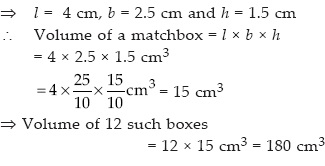Q 38.

A cuboidal water tank is 6 m long, 5 m wide and 4.5 m deep. How many litres of water can it hold∴ (1 m³=1000l)

SOLUTION:

Length (l)=6 m; Breadth (b)=5 m;
Depth (h)=4.5 m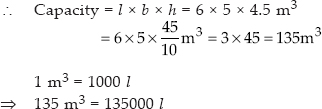∴ The required amount of water in the tank=135000 l.

Q 39.

A cuboidal vessel is 10 m long and 8 m wide. How high must it be made to hold 380 cubic metres of a liquid.

SOLUTION:

Length (l)=10 m; Breadth (b)=8 m
Volume (V)=380 m³
Let height of the cuboid be h
Since, volume of a cuboid=l x b x h
∴ Volume of the cuboidal vessel
= 10 x 8 x h m³=80h m³
∴ 80h=380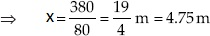Thus, the required height of the liquid=4.75 m

Q 40.

Find the cost of digging a cuboidal pit 8 m long, 6 m broad and 3 m deep at the rate of ₹30 per m³.

SOLUTION:

Length (l) = 8 m
Depth (h) = 3 m
∴ Volume of the cuboidal pit = l x b x h
= 8 x 6 x 3 m³ = 144 m³
Cost of digging = ₹ 144 x 30 = ₹ 4320

Q 41.

The capacity of a cuboidal tank is 50000 litres of water. Find the breadth of the tank, if its length and depth are respectively 2.5 m and 10 m.

SOLUTION:

Length of the tank (l)=2.5 m
Depth of the tank (h)=10 m
Let breadth of the tank=b m
∴ Volume (capacity) of the tank=l x b x h
= 2.5 x b x 10 m³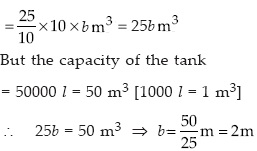Thus, the breadth of the tank=2m

Q 42.

A village, having a population of 4000, requires 150 litres of water per head per day. It has a tank measuring 20 m x 15 m x 6 m. For how many days will the water of this tank last.

SOLUTION:

Length of the tank (l)=20 m
Breadth of the tank (b)=15 m
Height of the tank (h)=6 m
∴ Volume of the tank=l x b x h
= 20 x 15 x 6 m³=1800 m³
Since 1 m³=1000 l
∴Capacity of the tank=1800 x 1000 l=1800000 l
Village population=4000
Since, 150 l of water is required per head per day.
∴ Amount of water required per day is=150 x 4000 l.
Let the required number of days='X'
∴ 4000 x 150 x X=1800000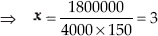Thus, the required number of days is 3.

Q 43.

A godown measures 40 m x 25 m x 10 m. Find the maximum number of wooden crates each measuring 1.5 m x 1.25 m x 0.5 m that can be stored in the godown.

SOLUTION:

Volume of the godown=40 x 25 x 10 m³
Volume of a crate=1.5 x 1.25 x 0.5 m³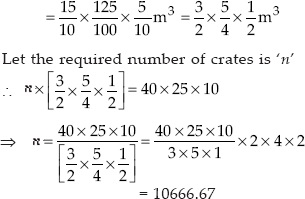∴ Maximum number of wooden crates=10667.

Q 44.

A solid cube of side 12 cm is cut into eight cubes of equal volume. What will be the side of the new cube. Also, find the ratio between their surface areas.

SOLUTION:

Side of the given cube=12 cm
∴ Volume of the given cube=(side)³
= (12)³ cm³
Let the side of the new (smaller) cube=n
∴ Volume of smaller cube=n³
∴ Volume of 8 smaller cubes=8n³
According to question,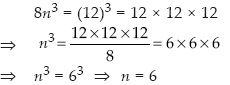Thus, the required side of the new (smaller) cube is 6 cm.
Now, Surface area of the given cube
= 6 x (side)²=6 x 12² cm²=6 x 12 x 12 cm²
Surface area of one smaller cube=6 x (side)²=6 x 6² cm²=6 x 6 x 6 cm²
Now,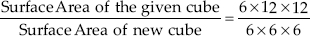= 4 : 1
Thus, the required ratio=4 : 1

Q 45.

A river 3 m deep and 40 m wide is flowing at the rate of 2 km per hour. How much water will fall into the sea in a minute

SOLUTION:

The water flowing in a river can be considered in the form of a cuboid.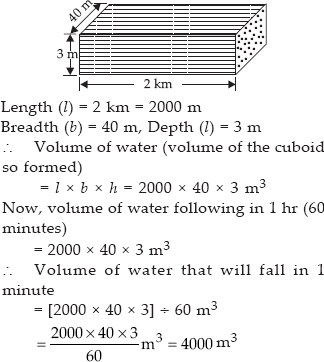Q 46.

The circumference of the base of a cylindrical vessel is 132 cm and its height is 25 cm. How many litres of water can it hold. (1000 cm³ = 1 litre)

SOLUTION:

Let the base radius of the cylindrical vessel be r cm.
∴ Circumference=2πr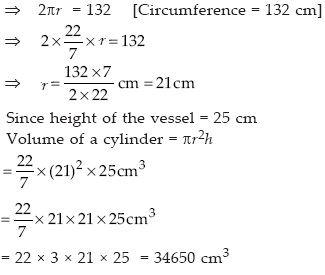Capacity of the vessel=Volume of the vessel
∴ Capacity of cylindrical vessel=34650 cm³
Since, 1000 cm³=1 litre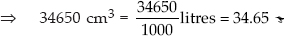Thus, the vessel can hold 34.65 l of water.

Q 47.

The inner diameter of a cylindrical wooden pipe is 24 cm and its outer diameter is 28 cm. The length of the pipe is 35 cm. Find the mass of the pipe, if 1 cm³ of wood has a mass of 0.6 g.

SOLUTION:

Inner diameter of the cylindrical pipe = 24 cm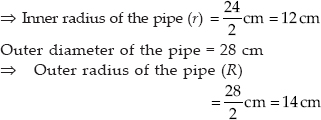Length of the pipe (h)=35 cm
Inner volume of the pipe=πr²h
Outer volume of the pipe=πR²h
∴ Amount of wood (volume) in the pipe
= Outer volume - Inner volume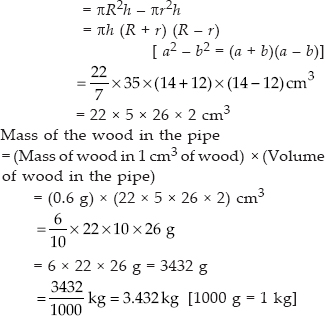Thus, the required mass of the pipe is 3.432 kg.

Q 48.

A soft drink is available in two packs : (i) a tin can with a rectangular base of length 5 cm and width 4 cm, having a height of 15 cm and (ii) a plastic cylinder with circular base of diameter 7 cm and height 10 cm.
Which container has greater capacity and by how much.

SOLUTION:

For rectangular pack
Length (l)=5 cm, Breadth (b)=4 cm
Height (h)=15 cm
Let height of the cuboid be 'h'
Volume=l x b x h=5 x 4 x 15 cm³=300 cm³
∴ Capacity of the rectangular pack
= 300 cm³
For cylindrical pack, Base diameter=7 cm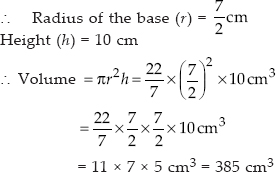∴ Volume of the cylindrical pack=385 cm³
So, the cylindrical pack has the greater capacity by 85 cm³.

Q 49.

If the lateral surface of a cylinder is 94.2 cm² and its height is 5 cm, then find
(ii) its volume. (Use π= 3.14)

SOLUTION:

Height of the cylinder (h)=5 cm Let the base radius of the cylinder be r (i) Since lateral surface of the cylinder=2πrh But lateral surface of the cylinder=94.2 cm²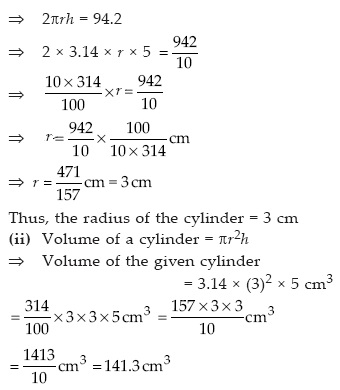Thus, the required volume=141.3 cm³

Q 50.

It costs ₹ 2200 to paint the inner curved surface of a cylindrical vessel 10 m deep. If the cost of painting is at the rate of ₹ 20 per m², find
(i) inner curved surface area of the vessel,
(iii) capacity of the vessel.

SOLUTION:

(i) Total cost of painting=₹ 2200
Cost of 1 m²=₹ 20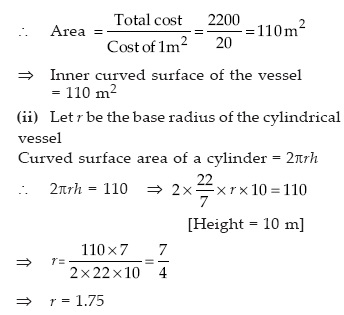∴ The required radius of the base=1.75 m
(iii) Since, volume of a cylinder=πr²h
∴ Volume (capacity) of the vessel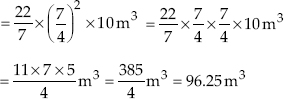Since, 1 m³=1000000 cm³=1000 l=1 kl
∴ 96.25 m³=96.25 kl
Thus, the required volume=96.25 kl

Q 51.

The capacity of a closed cylindrical vessel of height 1 m is 15.4 litres. How many square metres of metal sheet would be needed to make it.

SOLUTION:

Capacity of the cylindrical vessel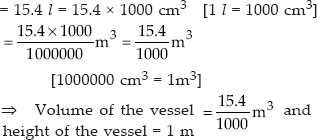Let r metres be the radius of the base of the vessel.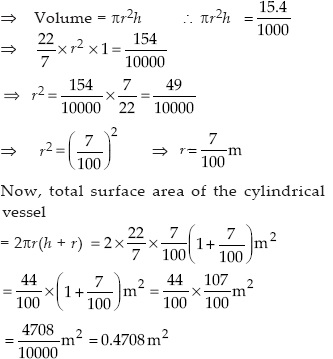Thus, the required sheet=0.4708 m².

Q 52.

A lead pencil consists of a cylinder of wood with a solid cylinder of graphite filled in the interior. The diameter of the pencil is 7 mm and the diameter of the graphite is 1 mm. If the length of the pencil is 14 cm, find the volume of the wood and that of the graphite.

SOLUTION: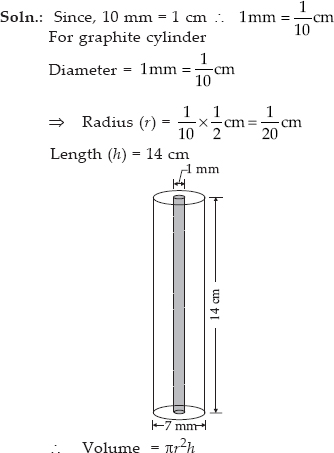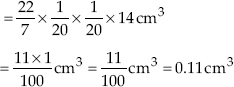Thus, the required volume of the graphite=0.11 cm³
Now, diameter of the pencil=7 mm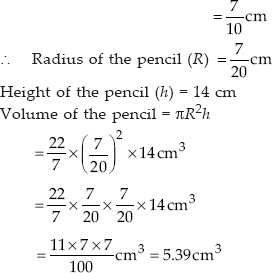Volume of the wood=[Volume of the pencil]
- [Volume of the graphite]
= 5.39 cm³ - 0.11 cm³=5.28 cm³
Thus, the required volume of the wood is 5.28 cm³

Q 53.

A patient in a hospital is given soup daily in a cylindrical bowl of diameter 7 cm. If the bowl is filled with soup to a height of 4 cm, how much soup the hospital has to prepare daily to serve 250 patients.

SOLUTION:

∵ The bowl is cylindrical, where diameter of the base=7 cm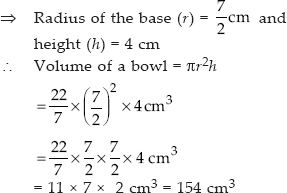i.e., Volume of soup in a bowl=154 cm³
∴ Volume of soup in 250 bowls
= 250 x 154 cm³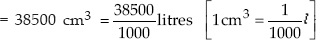Thus, the hospital needs to prepare 38.5 litres of soup daily for 250 patients.

Q 54.

Find the volume of the right circular cone with
(i) radius 6 cm, height 7 cm
(ii) radius 3.5 cm, height 12 cm

SOLUTION:

(i) Radius of the cone (r)=6 cm
Height (h)=7 cm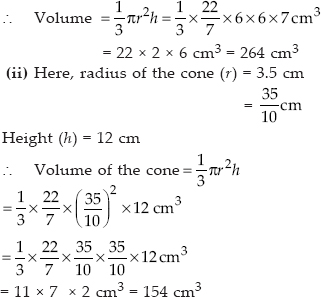Q 55.

Find the capacity in litres of a conical vessel with
(i) radius 7 cm, slant height 25cm
(ii) height 12 cm, slant height 13 cm

SOLUTION:

(i) Here, r=7 cm and l=25 cm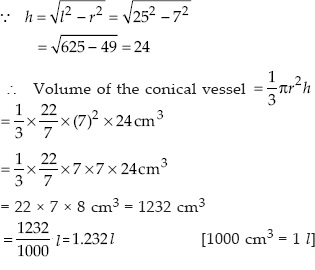Thus, the required capacity of the conical vessel is 1.232 l
(ii) Here, height (h)=12 cm and l=13 cm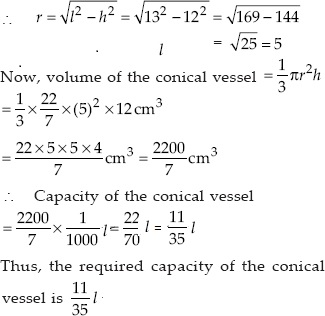Q 56.

The height of a cone is 15 cm. If its volume is 1570 cm³, find the radius of the base.
(Use π=3.14)

SOLUTION:

Here, height of the cone (h)=15 cm
Volume of the cone=1570 cm³
Let the radius of the base be r cm.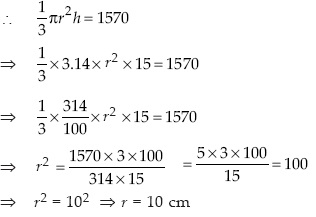Thus, the required radius of the base is 10 cm.

Q 57.

If the volume of a right circular cone of height 9 cm is 48 cm³, find the diameter of its base.

SOLUTION:

Volume of the cone=48 cm³
Height of the cone (h)=9 cm
Let 'r' be its base radius.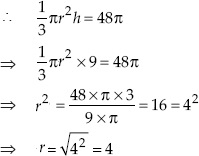∴ Diameter of the base of the cone=2 x 4 cm
= 8 cm

Q 58.

A conical pit of top diameter 3.5 m is 12 m deep. What is its capacity in kilolitres.?

SOLUTION:

Here, diameter of the conical pit=3.5 m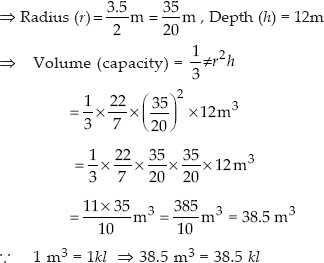Thus, the capacity of the conical pit is 38.5 kl.

Q 59.

The volume of a right circular cone is 9856 cm³. If the diameter of the base is 28 cm, find
(i) height of the cone
(ii) slant height of the cone
(iii) curved surface area of the cone

SOLUTION:

Volume of the cone=9856 cm³
Diameter of the base=28 cm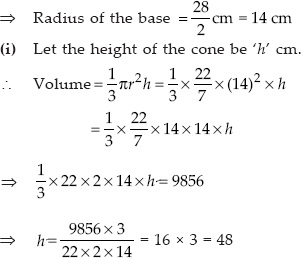Thus, the required height is 48 cm.
(ii) Let the slant height be 'l' cm.
∴ l²=14² + 48²=196 + 2304=2500=(50)²
∴ l=50

Thus, the required slant height=50 cm.

(iii) The curved surface area of a cone is given by rl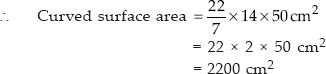Thus, the curved surface area of the cone is 2200 cm².

Q 60.

A right triangle ABC with sides 5 cm, 12 cm and 13 cm is revolved about the side 12 cm. Find the volume of the solid so obtained.

SOLUTION:

Sides of the right triangle are 5 cm, 12 cm and 13 cm.
The right angled triangle is revolved about the side 12 cm.
∴ Its height is 12 cm and base is 5 cm.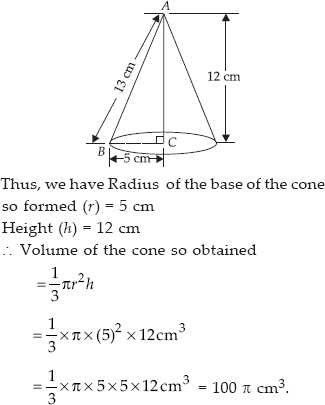Thus, the required volume of the cone is 100 πcm³.

Q 61.

If the triangle ABC in the Question 7 above is revolved about the side 5 cm, then find the volume of the solid so obtained. Find also the ratio of the
volumes of the two solids obtained in Questions 7 and 8.

SOLUTION:

Since the right triangle is revolved about the side 5 cm.
∴ Height of the cone so obtained (h)=5 cm
Radius of the cone (r)=12 cm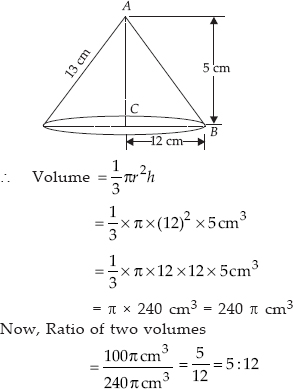Thus, the required ratio is 5 : 12.

Q 62.

A heap of wheat is in the form of a cone whose diameter is 10.5 m and height is 3m. Find its volume. The heap is to be covered by canvas to protect it from rain.
Find the area of the canvas required.

SOLUTION:

Here the heap of wheat is in the form of a cone with base diameter=10.5 m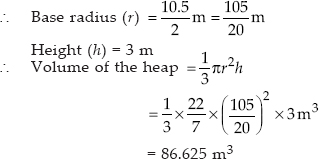Thus, the required volume=86.625 m³
Now the area of the canvas to cover the heap must equal to the curved surface area of the conical heap.
∴ Area of the canvas=rl,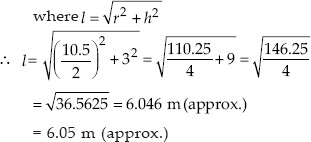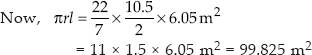Thus, the required area of the canvas is 99.825 m².

Q 63.

Find the volume of a sphere whose radius is
(i) 7 cm
(ii) 0.63 m

SOLUTION: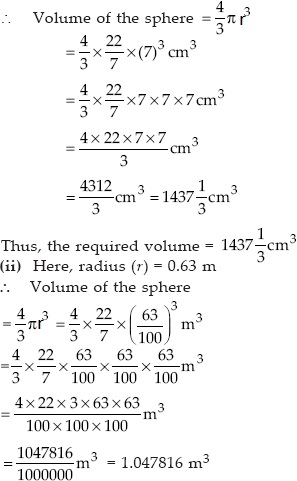Thus, the required volume is 1.05 m³ (approx.)

Q 64.

Find the amount of water displaced by a solid spherical ball of diameter
(i) 28 cm
(ii) 0.21 m

SOLUTION:

(i) Diameter of the ball=28 cm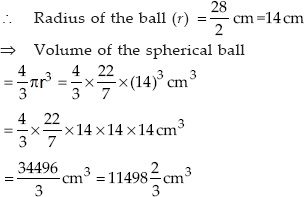Thus, the amount of water displaced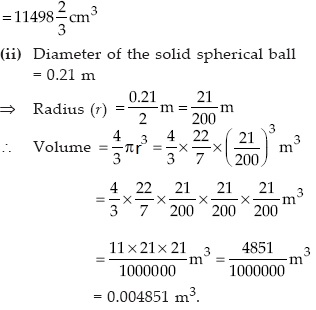Q 65.

The diameter of a metallic ball is 4.2 cm. What is the mass of the ball, if the density of the metal is 8.9 g per cm³.

SOLUTION:

Diameter of the metallic ball=4.2 cmThus, the mass of ball is 345.39 g (approx.)

Q 66.

The diameter of the moon is approximately one-fourth of the diameter of the earth. What fraction of the volume of the earth is the volume of the moon.

SOLUTION:

Let diameter of the earth be 2r.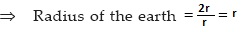Diameter of the moon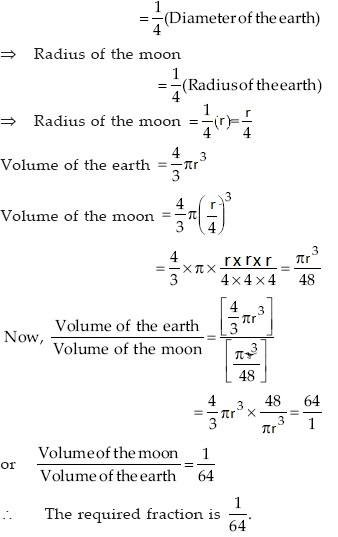Q 67.

How many litres of milk can a hemispherical bowl of diameter 10.5 cm hold.

SOLUTION:

Diameter of the hemisphere=10.5 cm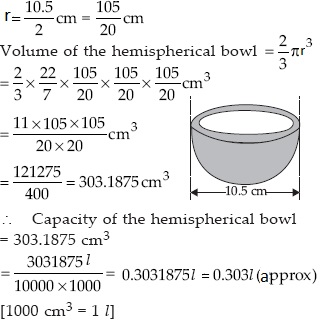Thus, the capacity of the bowl=0.303 l (approx.)

Q 68.

A hemispherical tank is made up of an iron sheet 1 cm thick. If the inner radius is 1 m, then find the volume of the iron used to make the tank.

SOLUTION: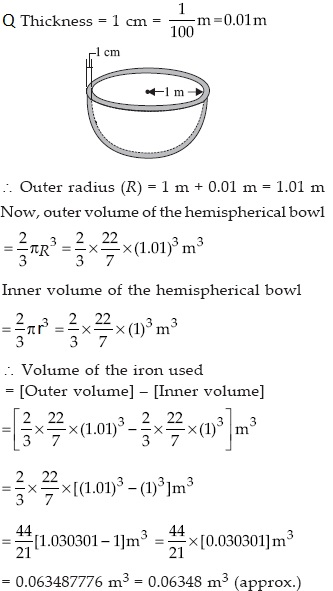Thus, the required volume of the iron=0.06348 m³

Q 69.

Find the volume of a sphere whose surface area is 154 cm².

SOLUTION:

Let 'r' be the radius of the sphere.
∴ Its surface area=4πr²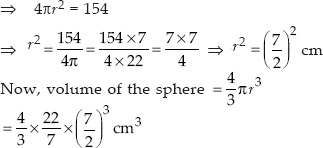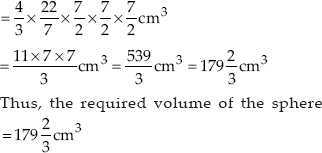Q 70.

A dome of a building is in the form of a hemisphere. From inside, it was white-washed at the cost of ₹498.96. If the cost of white-washing is ₹2.00 per square metre, find the
(i) inside surface area of the dome.
(ii) volume of the air inside the dome.

SOLUTION:

(i) Inside surface area of the dome
Total cost of white washing=₹498.96
Cost of 1m² of white washing=₹ 2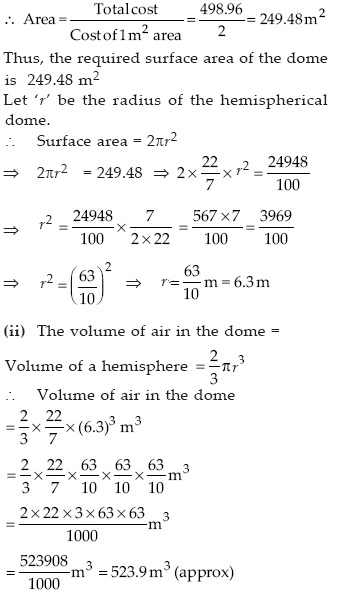Thus, the required volume of air inside the dome is 523.9 m³ (approx).

Q 71.

Twenty seven solid iron spheres, each of radius r and surface area S are melted to form a sphere with surface area S'. Find the
(i) radius r' of the new sphere,
(ii) ratio of S and S'.

SOLUTION:

(i) Let the radius of a small sphere=r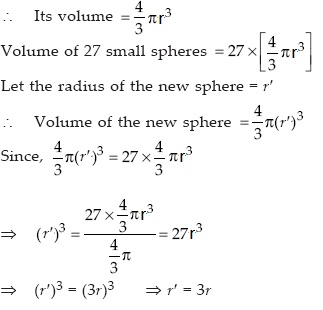(ii) Surface area of a small sphere=4r²
⇒ S=4r² and S′=4(3r)² [∵ r'=3r]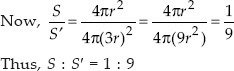Q 72.

A capsule of medicine is in the shape of a sphere of diameter 3.5 mm. How much medicine (in mm³) is needed to fill this capsule.

SOLUTION:

Diameter of the spherical capsule=3.5 mm
⇒ Radius of the spherical capsule (r)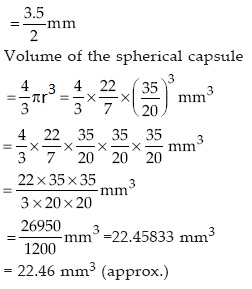Thus, the required quantity of medicine
= 22.46 mm³ (approx).